Ex 12.1

Chapter 12 Class 7 Symmetry
Serial order wise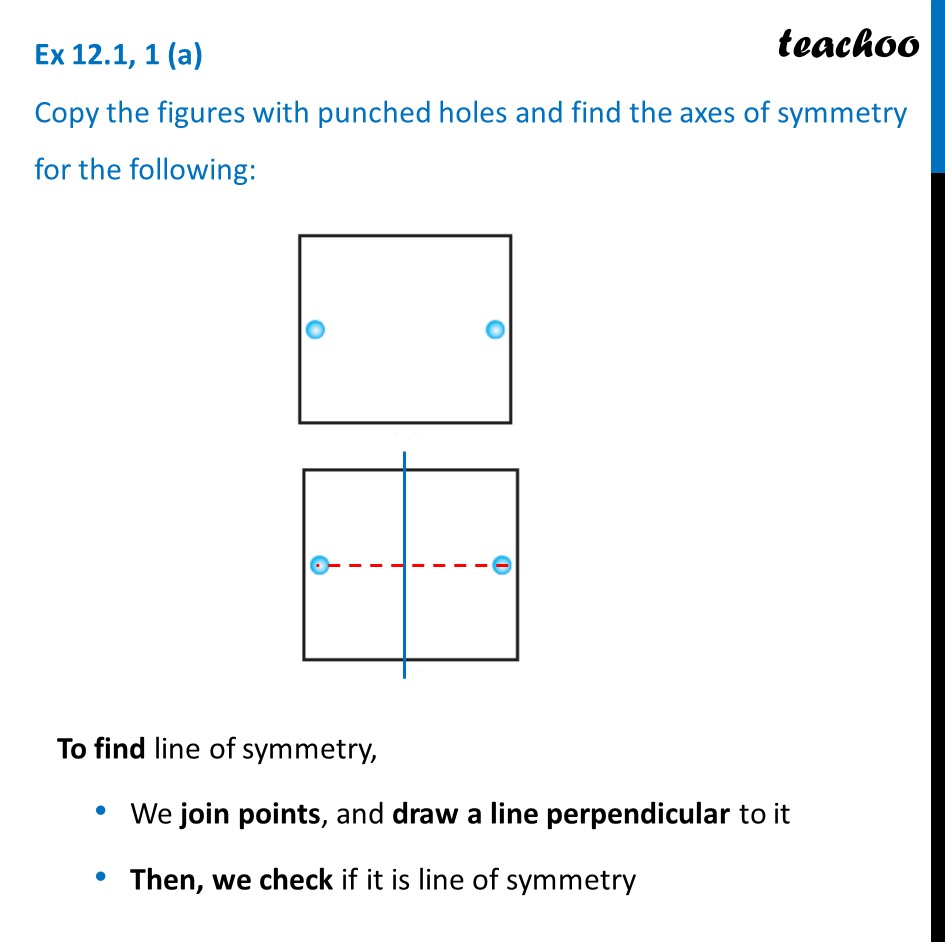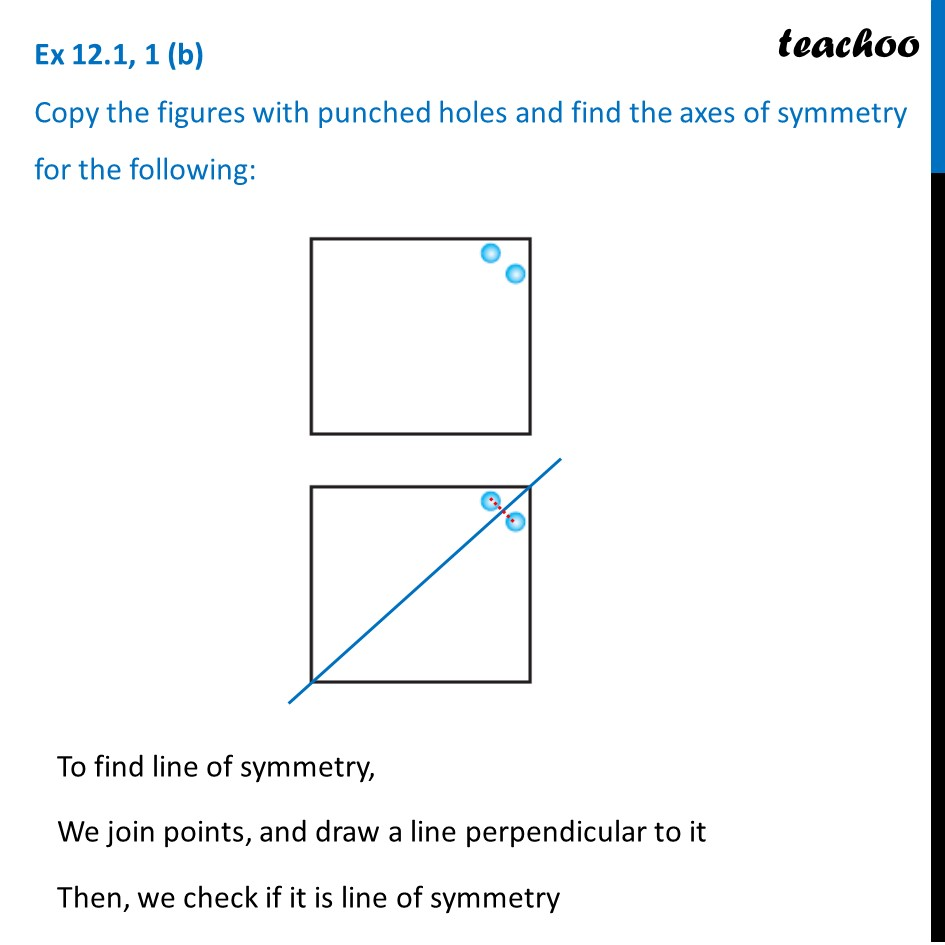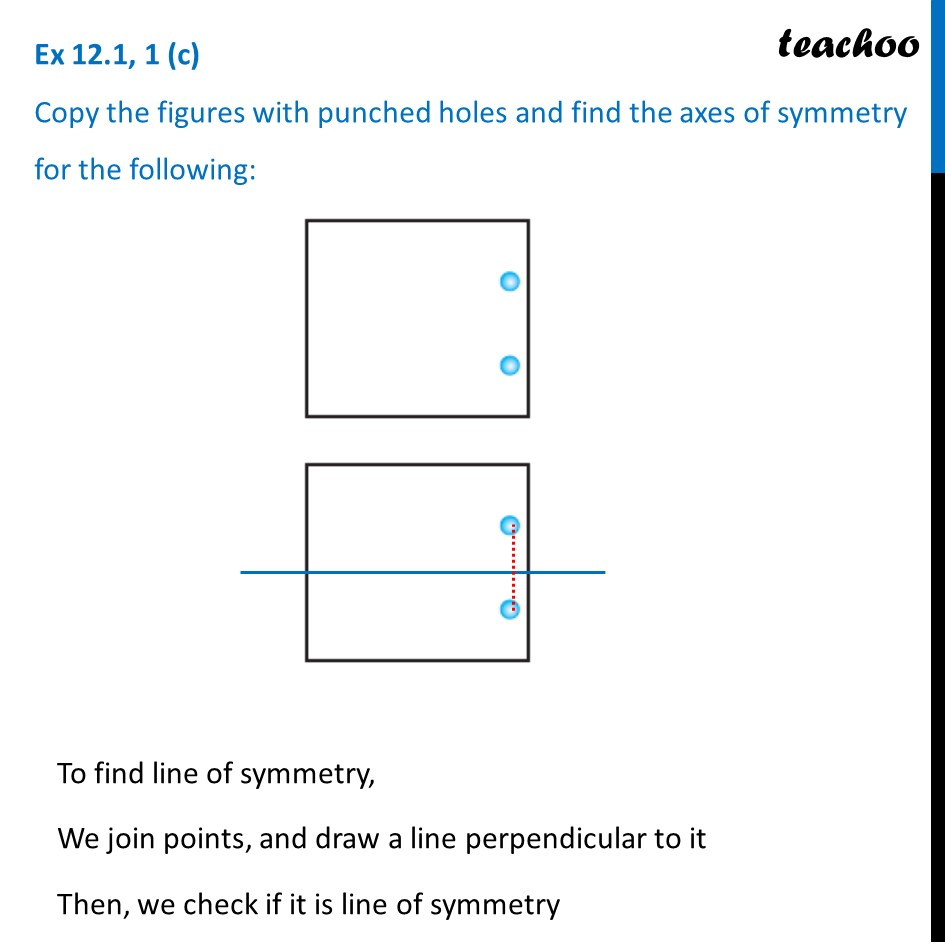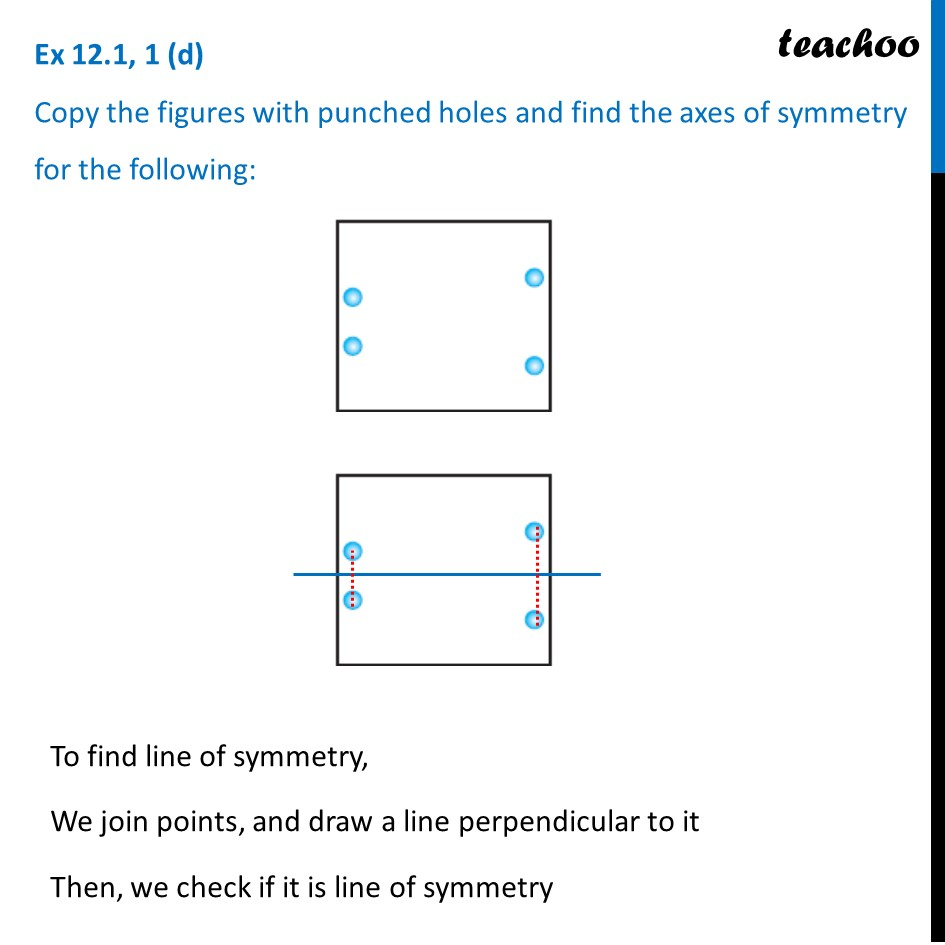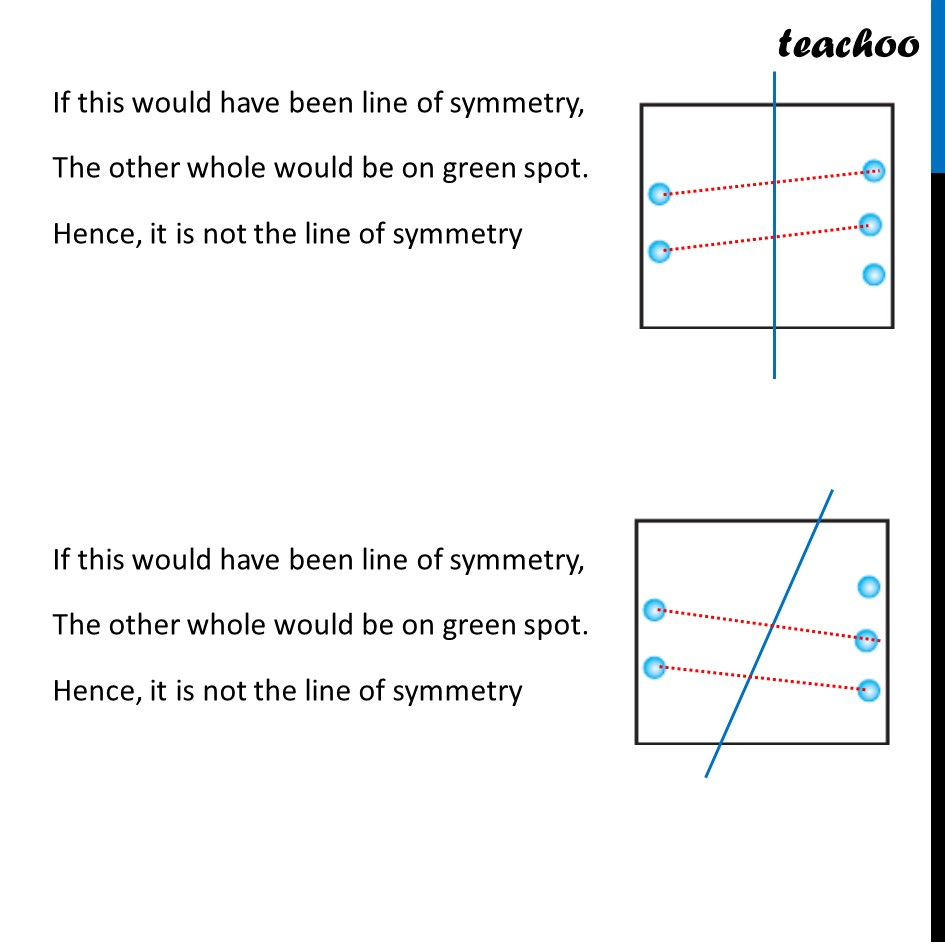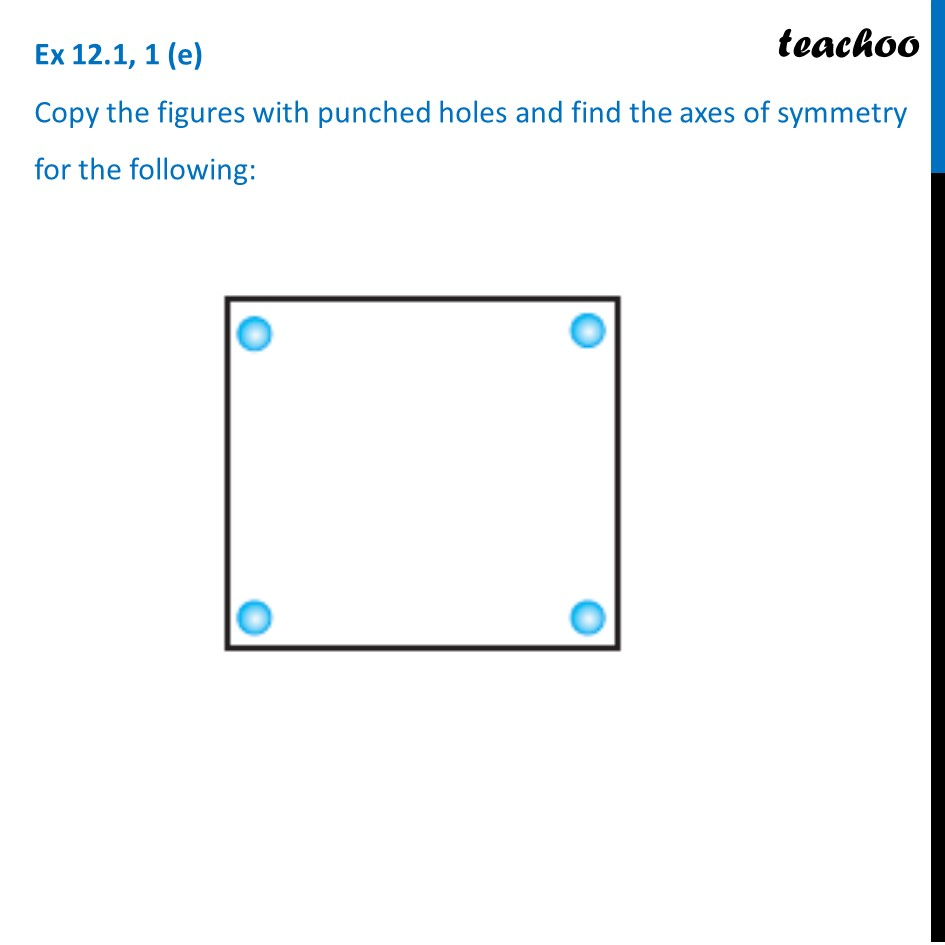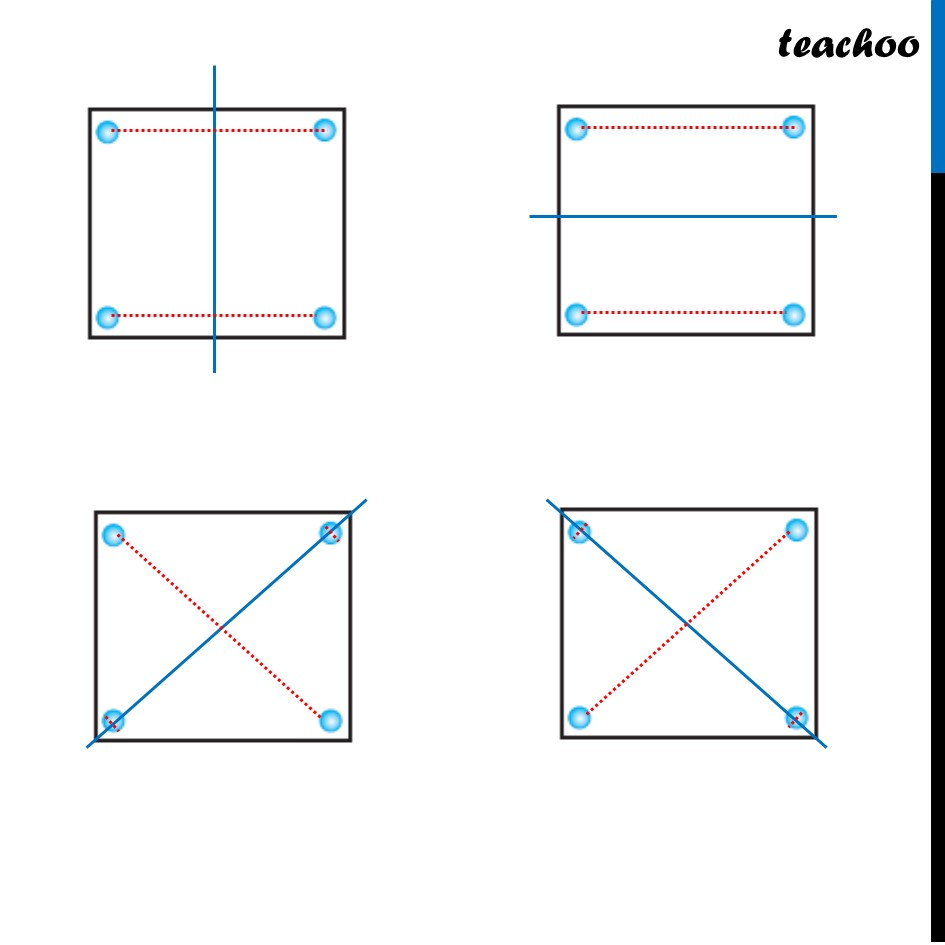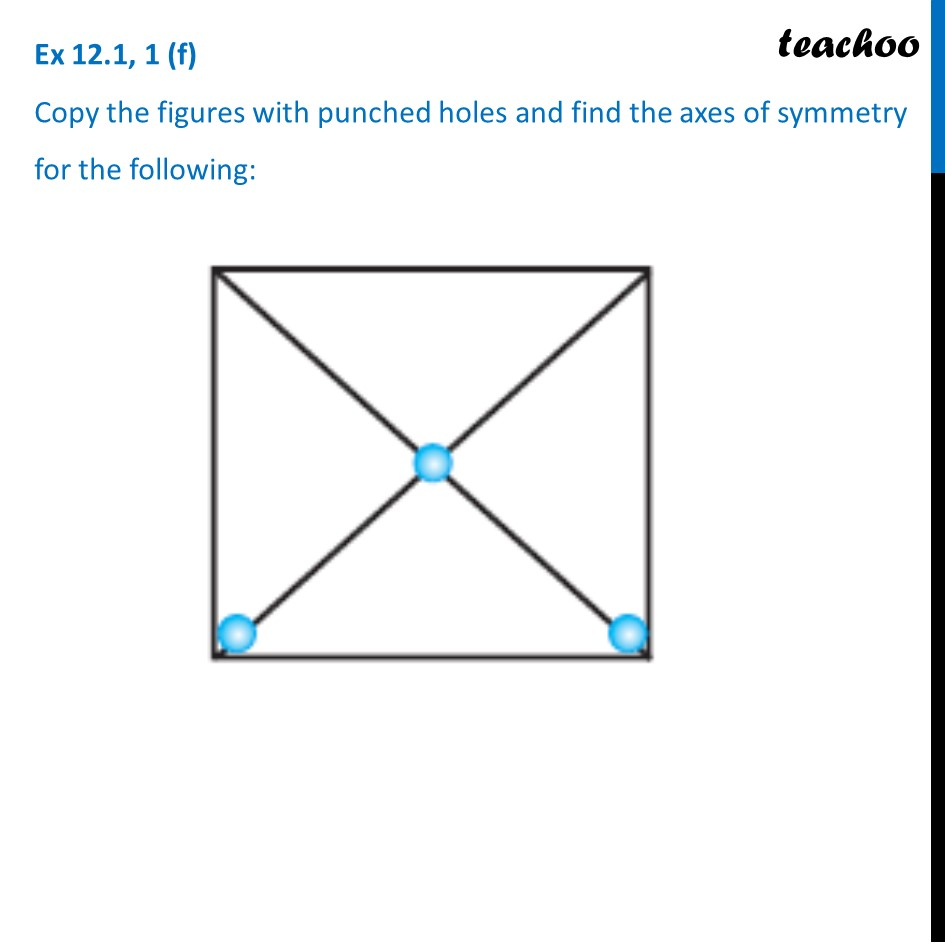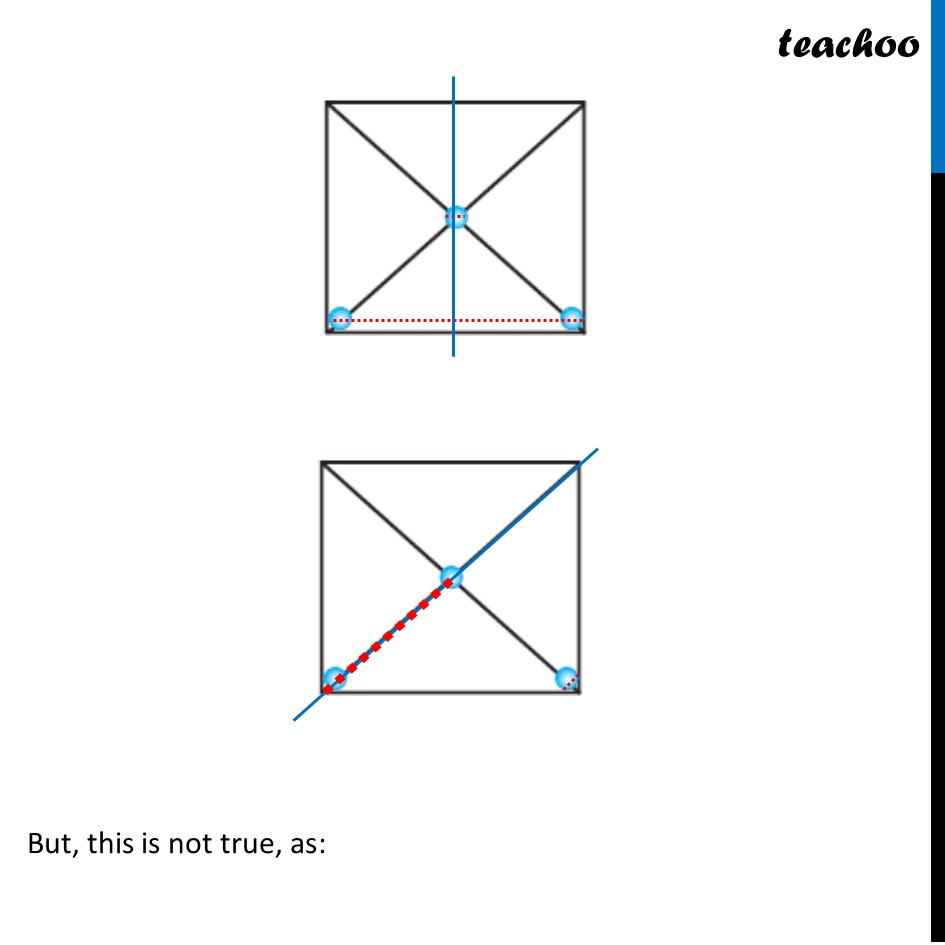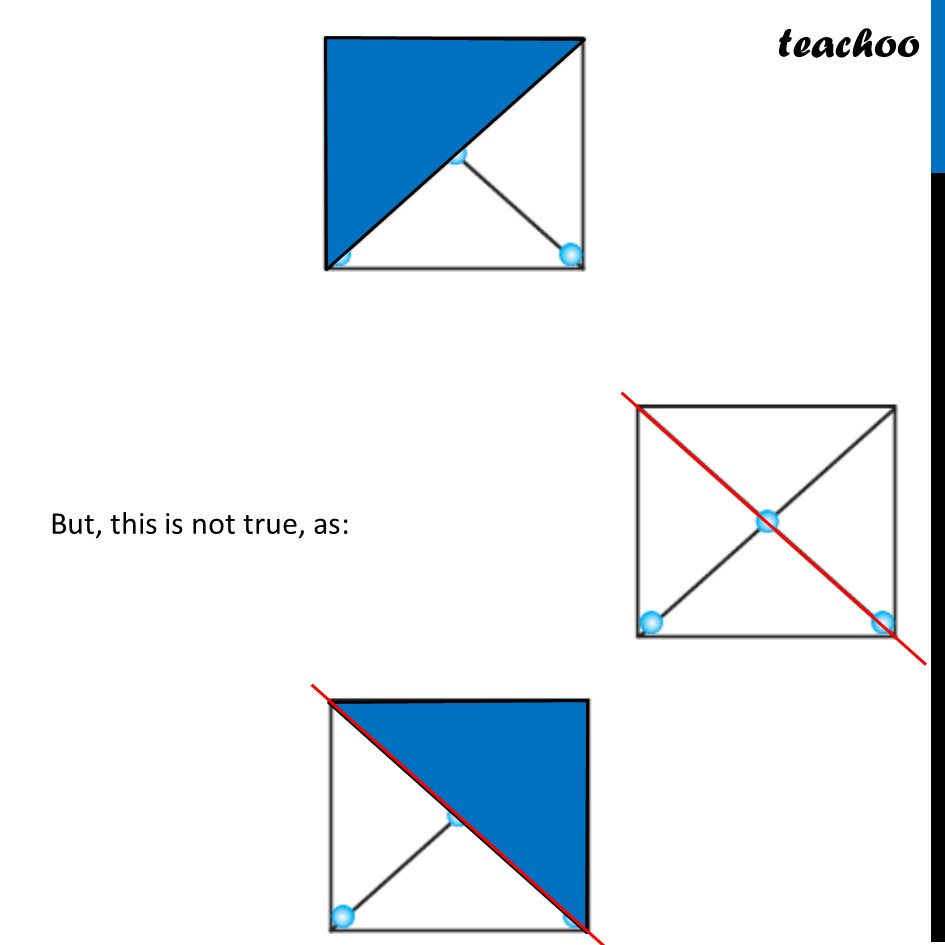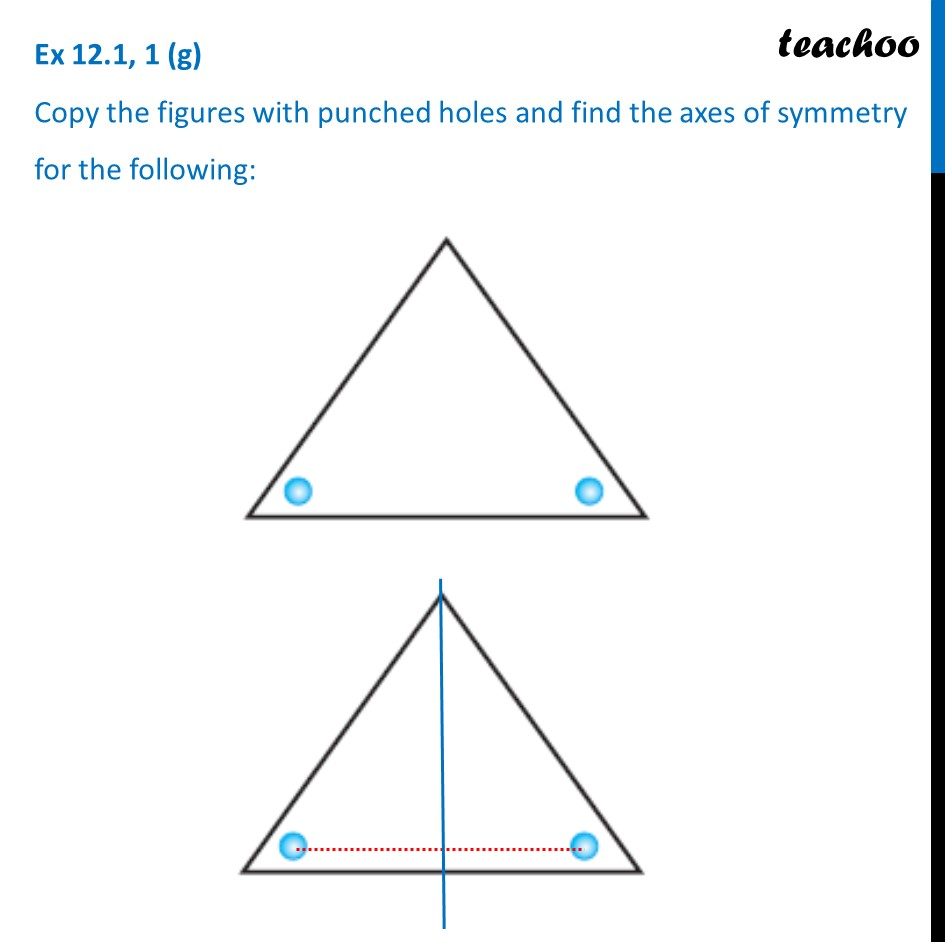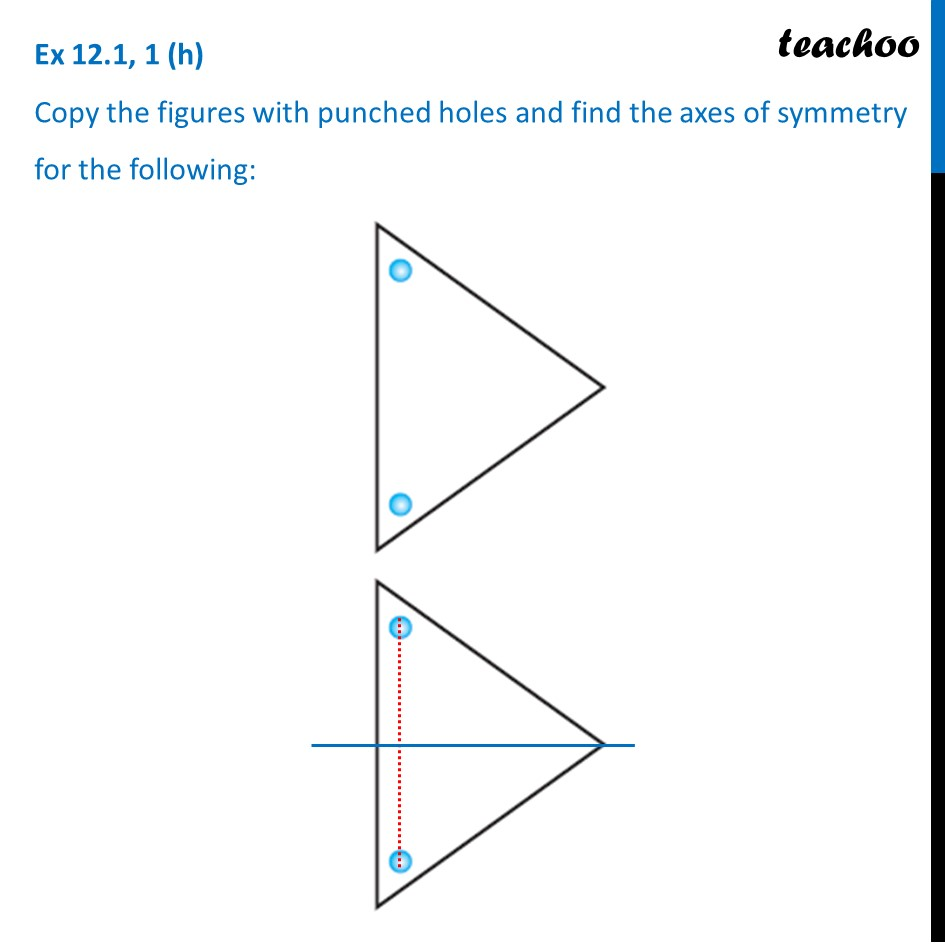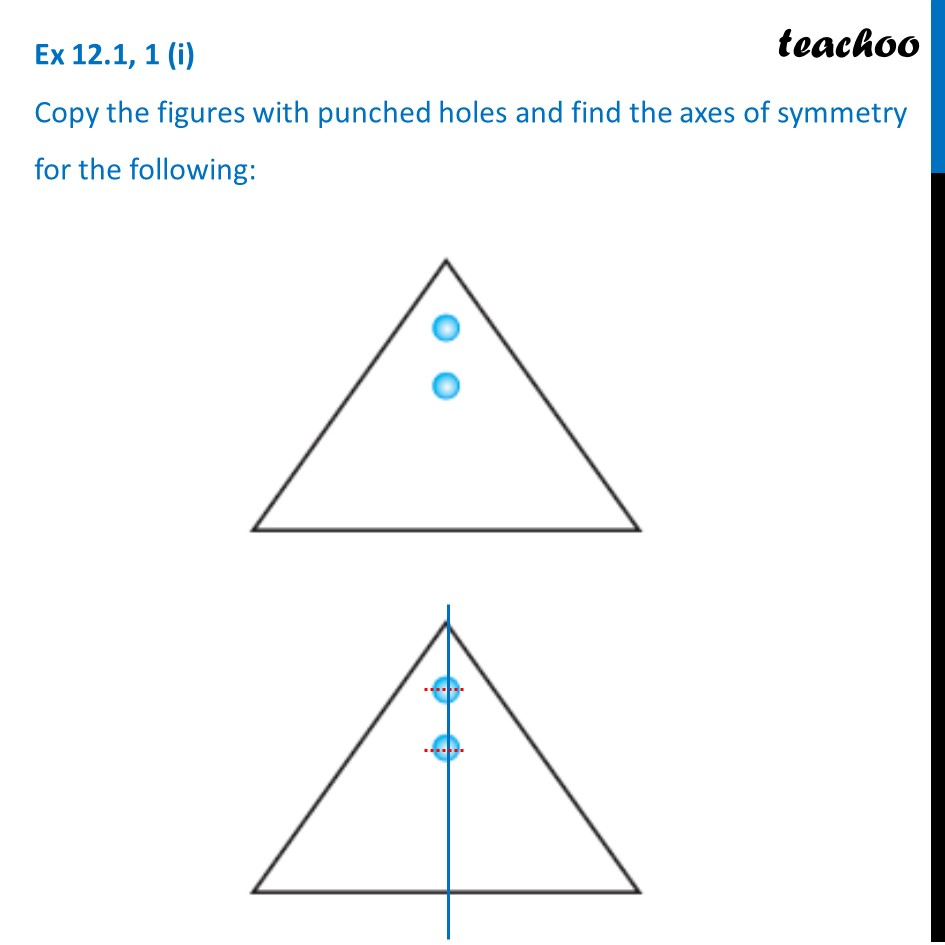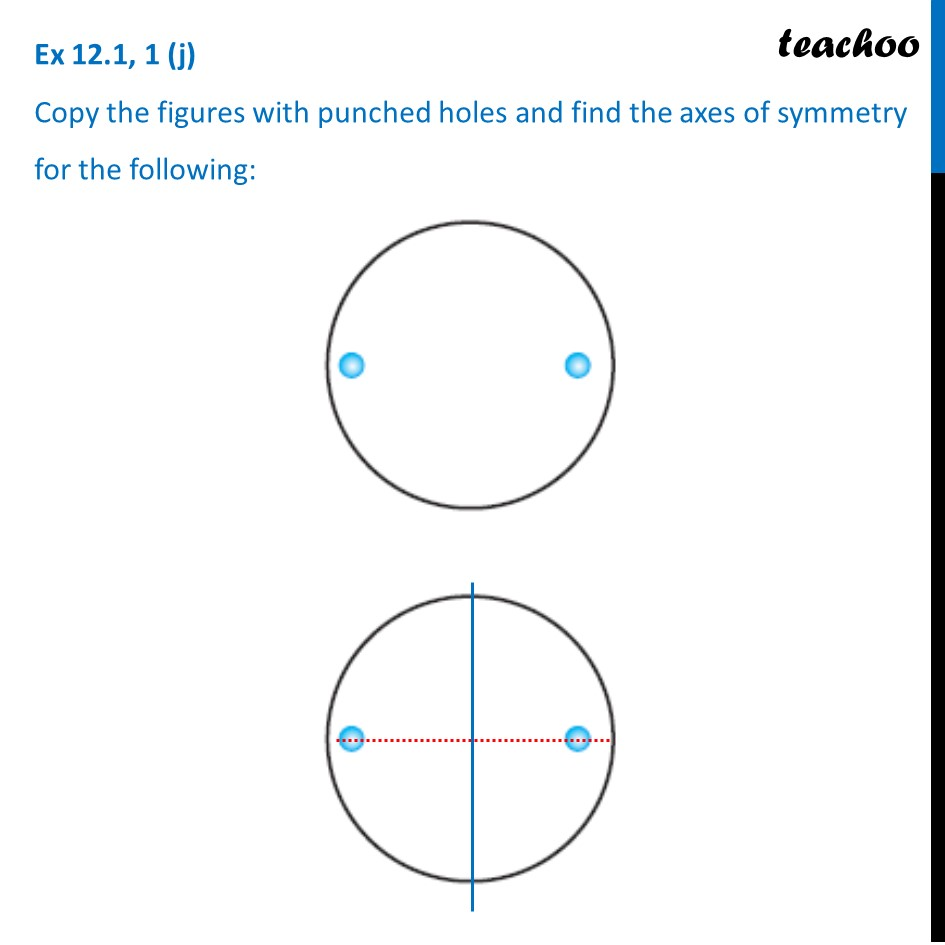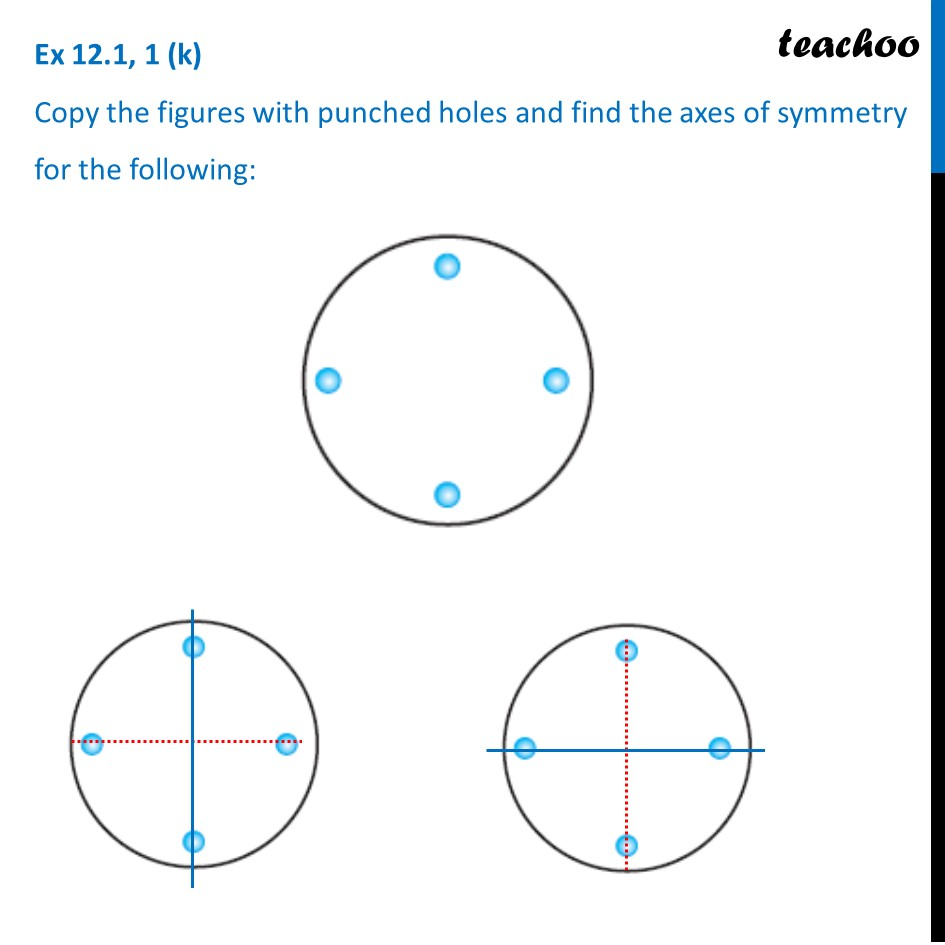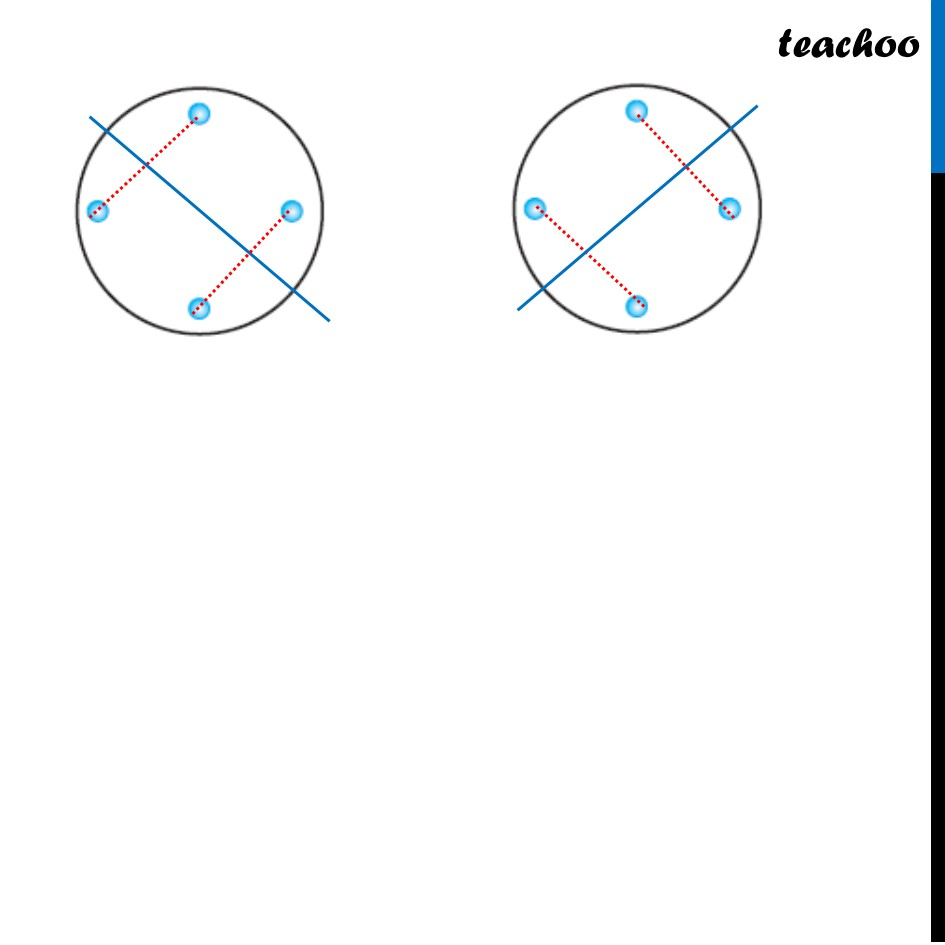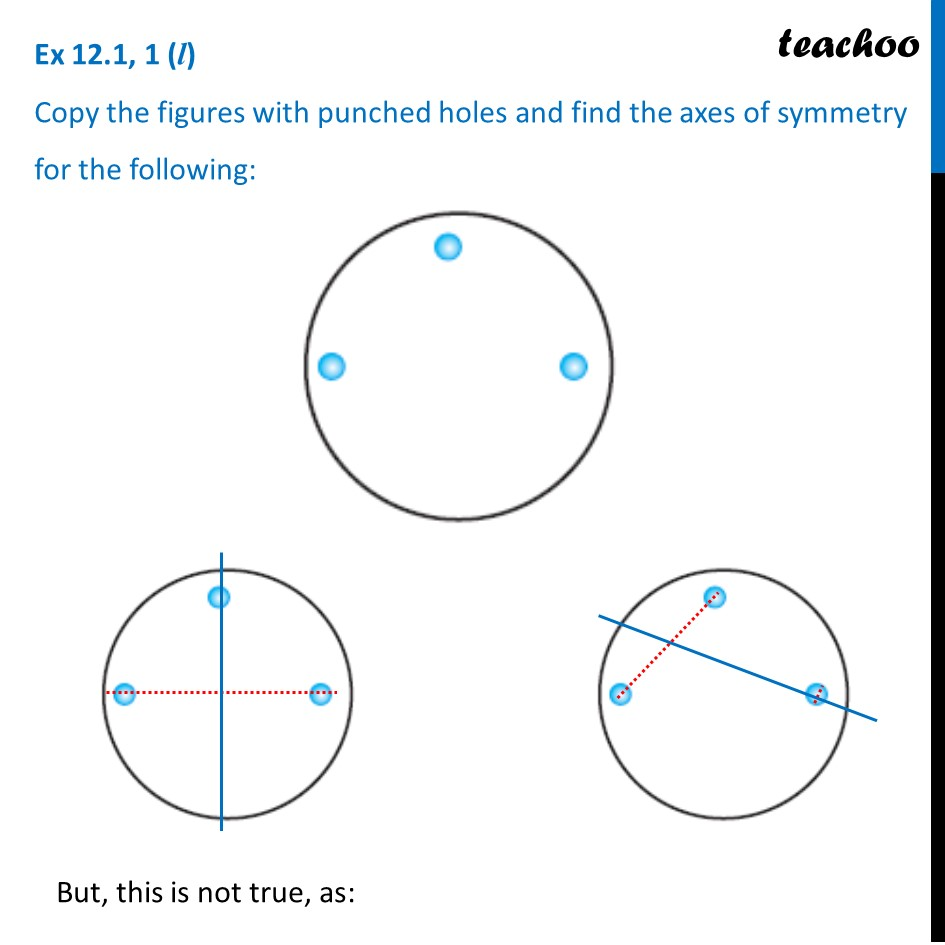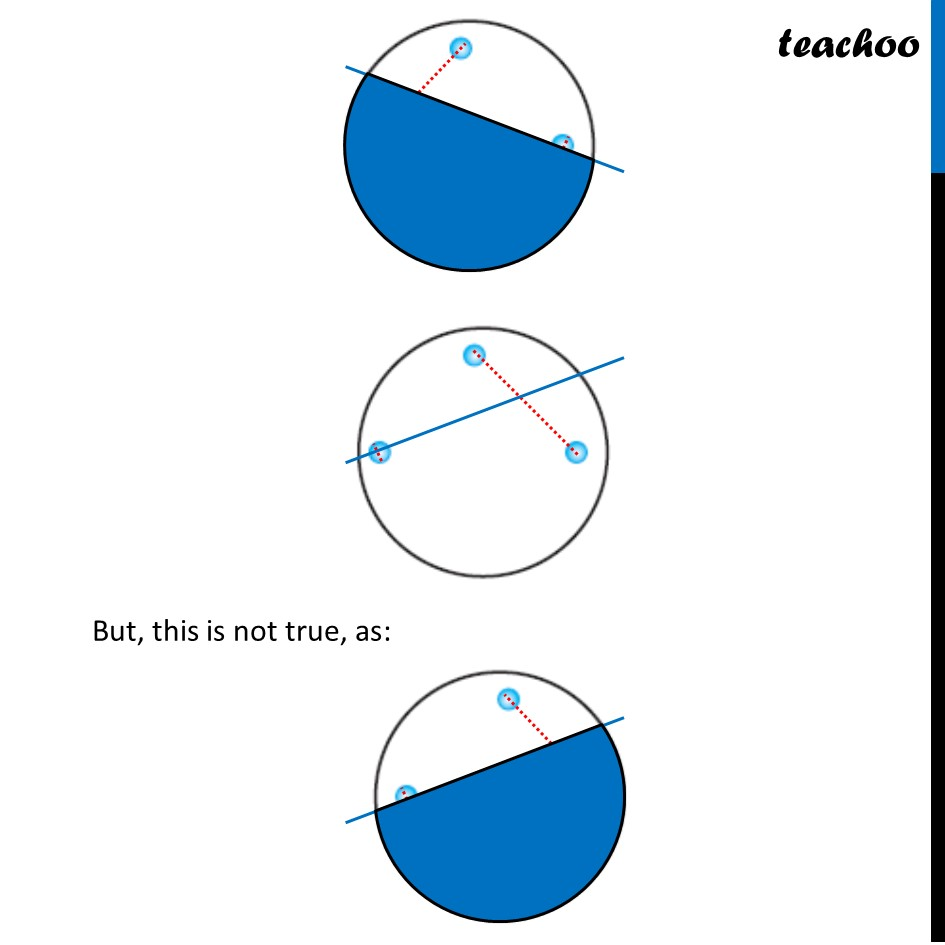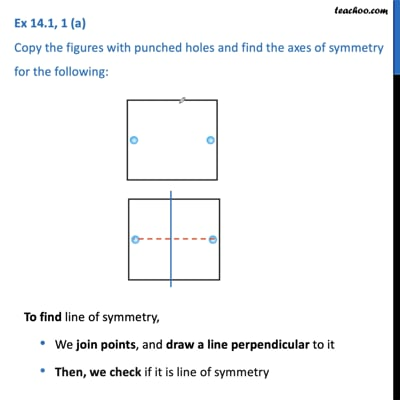This video is only available for Teachoo black users

Learn in your speed, with individual attention - Teachoo Maths 1-on-1 Class

### Transcript

Ex 12.1, 1 (a) Copy the figures with punched holes and find the axes of symmetry for the following: To find line of symmetry, We join points, and draw a line perpendicular to it Then, we check if it is line of symmetry Ex 12.1, 1 (b) Copy the figures with punched holes and find the axes of symmetry for the following: To find line of symmetry, We join points, and draw a line perpendicular to it Then, we check if it is line of symmetry Ex 12.1, 1 (c) Copy the figures with punched holes and find the axes of symmetry for the following: To find line of symmetry, We join points, and draw a line perpendicular to it Then, we check if it is line of symmetry Ex 12.1, 1 (d) Copy the figures with punched holes and find the axes of symmetry for the following: To find line of symmetry, We join points, and draw a line perpendicular to it Then, we check if it is line of symmetry If this would have been line of symmetry, The other whole would be on green spot. Hence, it is not the line of symmetry If this would have been line of symmetry, The other whole would be on green spot. Hence, it is not the line of symmetry Ex 12.1, 1 (e) Copy the figures with punched holes and find the axes of symmetry for the following:Ex 12.1, 1 (f) Copy the figures with punched holes and find the axes of symmetry for the following: Ex 12.1, 1 (g) Copy the figures with punched holes and find the axes of symmetry for the following: Ex 12.1, 1 (h) Copy the figures with punched holes and find the axes of symmetry for the following: Ex 12.1, 1 (i) Copy the figures with punched holes and find the axes of symmetry for the following: Ex 12.1, 1 (j) Copy the figures with punched holes and find the axes of symmetry for the following: Ex 12.1, 1 (k) Copy the figures with punched holes and find the axes of symmetry for the following: Ex 12.1, 1 (l) Copy the figures with punched holes and find the axes of symmetry for the following: But, this is not true, as: